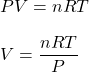Question

The volume of a 1.00-mole sample of an ideal gas will decrease when the
1.
pressure decreases and the temperature decreases
2.
pressure decreases and the temperature increases
3.
pressure increases and the temperature decreases
4.
pressure increases and the temperature increases

1.thuthao

The correct option is (3).

Explanation:

The ideal gas equation is given by :The pressure of ideal gas is directly proportional to temperature and inversely proportional to its volume.

We can say that the volume of a 1.00-mole sample of an ideal gas will decrease when the pressure increases and the temperature decreases.